# I have no clue how to interview for data scientists

At work we have been expanding quite a bit, so this comes with many interviews for data science candidates (as well as MLOps and a few business analysts). For a bit while we were between directors, I did the initial screening interviews (soft questions, get some background, just filter out those really out of depth). I filtered very few people in the end, and I have no clue if that was good/bad. One of the hard things about interviews is it is a very lossy feedback loop. You only really know if it worked out well 6+ months after it is over. And you only get on-policy feedback for those you hired – you don’t know if you filtered out someone who would have worked really well.

I have done more of the technical interviews though recently, which are intended to be more discriminatory. I don’t believe I do a good job at this either, or at least everyone seems OK (no clear uber bad and no clear uber good). It has been particularly hard to hire people who can come in and be seniors/independent from the get-go, as even people with many years of experience it isn’t clear to me they have the right experience to be really independent after on-boarding for a month.

For a while we did the homework thing – here is a dataset, do some data manipulation and fit a model. (You can see the homework I made on GitHub, longer version, shorter version.) We have stopped doing these though, partly because everyone’s homework looks the same – what I call copy-pasta code. So I feel asking people to spend 4-8 hours (or likely more) on homework is not good for the very little benefit it provides. The nature of simple homework assignments I believe is so superficial I don’t think you can do it in a way that is amenable to anything but copy-pasta.

So now during the technical interview we do a grilling of questions. We have some regulars, but they do not appear to me to be really discriminatory either.

So I typically start by asking people to pick a project they think had the most value, and we do a deep dive into that. Many people who are good programmers (and some even with math degrees) don’t have what I would consider real fundamental knowledge of the models they are building. How many parameters do you have in your deep learning model? Because you oversampled how did you recalibrate the predictions to correctly estimate false positives? How did you evaluate the return on investment to your model? I feel these are quite fair – I let you pick the best work you have done in your career! You should be quite familiar with it.

So now that I am unsure if you should be left alone to build models, we migrate to some specific technical questions. These can be explain the difference between random forests and xgboost models, explain an ROC curve to a business person, what is the difference between a list and a tuple in python, if you had to query a million claims once a week and apply a predictive model how would you do it, etc. (Tend to focus more on math/stats than programming.)

Those examples above most people answer just fine (so they are maybe worthless, they don’t discriminate anyone). But a few pretty much everyone we interview fails – one is this question about calculating expected utility for auditing claims with a different dollar value amount I shared on LinkedIn the other day:

Pretend you have a model that predicts the probability an insurance claim is fraudulent. You have two claims; one \$2000 with a 50% probability, and one \$10000 with a 20% probability. If you had to choose a single claim to audit, which would one would you pick and why?

Am I crazy to expect someone with a data science degree to be able to answer this question coherently? (Pretty close to every model we have focusing on health insurance claims at Gainwell looks like this in real life! It is not a weird, unrealistic scenario.) I have more generic variants as well, such as how do you take a predicted probability and a claim value and know it is worth it to audit. Or for a more generic one how do you know how to set the threshold to audit claims given a model prediction? These questions appear too discriminatory, in that they filter out even very experienced individuals.

This and the threshold question kills me a little inside everytime a senior person mangles the logic of it – it really signals to me you don’t understand how to translate mathematical models to make relevant business decisions. To be an independent data scientist this is a critical skill – you need it to know how to structure the model as well as how to feed that back into whatever human or automated decision making process uses that models predictions. It is what distinguishes data scientists from software engineers – I am an applied mathematician who knows how to code is how I view my role. (It is one of the reasons I think a PhD is valuable – being able to think mathematically like that in broader strokes is a typical step in the dissertation process for folks.)

So I am stuck between questions everyone can answer and questions no one can answer. I feel like I might as well flip a coin after the initial entry level interview and not waste everyone’s time.

# Simulating runs of events

I still lurk on the Cross Validated statistics site every now and then. There was a kind of common question about the probability of a run of events occurring, and the poster provided a nice analytic solution to the problem using Markov Chains and absorbing states I was not familiar with.

I was familiar with a way to approximate the answer though using a simple simulation, and encoding data via run length encoding. Run length encoding works like this, if you have an original sequence that is `AABBBABBBB`, then the run length encoded version of this sequence is:

``````A,2
B,2
A,1
B,4``````

This is a quite convenient sparse data format to be familiar with. E.g. if you are using tensors in various deep learning libraries, you can encode the data like this and then stack the tensor. But the stacked tensor is just a view, so it doesn’t take up as much memory as the initial full tensor.

Using this encoding also makes a simulation to answer the question, how often do runs of 5+ occur in this hypothetical experiment quite easy to estimate. You just calculate the run length encoded version of the data, and see if any of the lengths are equal to or greater than 5. Below are code snippets in R and Python.

While the analytic solution is of course preferable when you can figure it out, simulations are nice to test whether the solution is correct, as well as to provide an answer when you are not familiar with how to analytically derive a solution.

# R Code

R has a native run-length encoding command, `rle`. The reason is that runs tests are a common time series technique for looking at randomness. Encourage you to run the code yourself to see how my simulated answer lines up with the analytic answer provided on the stats site!

``````##########################################
# R Code
set.seed(10)
die <- 1:6
run_sim <- function(rolls=1000, conseq=5){
test <- sample(die,rolls,TRUE)
res <- max(rle(test)\$lengths) >= conseq
return(res)
}

sims <- 1000000
results <- replicate(sims, run_sim(), TRUE)
print( mean(results) )
##########################################``````

# Python Code

The python code is very similar to the R code. Main difference is there is no native run length encoding command in numpy or scipy I am aware of (although there should be)! So I edited a function I found from Stackoverflow to accomplish the rle.

``````##########################################
# Python code

import numpy as np
np.random.seed(10)

# Edited from https://stackoverflow.com/a/32681075/604456
# input numpy arrary, return tuple (lengths, vals)
def rle(ia):
y = np.array(ia[1:] != ia[:-1])         # pairwise unequal (string safe)
i = np.append(np.where(y), len(ia) - 1) # must include last element
z = np.diff(np.append(-1, i))           # run lengths
return (z, ia[i])

die = list(range(6))

def run_sim(rolls=1000, conseq=5):
rlen, vals = rle(np.random.choice(a=die,size=rolls,replace=True))
return rlen.max() >= conseq

sims = 1000000
results = [run_sim() for i in range(sims)]
print( sum(results)/len(results) )
##########################################``````

I debated on expanding this post to show how to do these simulations in parallel, this is a bit of a cheesy experiment to show though. To do 1 million simulations on my machine still only takes like 10~20 seconds for each of these code snippets. So that will have to wait until another post!

You may be thinking why do I care about runs of dice rolls? Well, it can be extended to many different types of time series monitoring problems. For example, when I worked as a crime analyst at Troy I thought about this in terms of analyzing domestic violent reports. They were too numerous for me to read through every report, so I needed to devise a system to identify if there were anomalous patterns in the recent number of reports. You could devise a test here, say how many days of 10+ reports in a row, and see how frequently you would expect that occur in say a year of monitoring. The simulations above could easily be amended to do that, via doing simulations of the Poisson distribution instead of dice rolls, or assigning weights to particular outcomes.

# Some notes on PChange – estimating when trajectories cross over time

J.C. Barnes and company published a paper in JQC not too long ago and came up with a metric, PChange, to establish the number of times trajectories cross in a sample. This is more of interest to life course folks, although it is not totally far fetched to see it applied to trajectories of crime at places. Part of my interest in it was simply that it is an interesting statistical question — when two trajectories with errors cross. A seemingly simple question that has a few twists and turns. Here are my subsequent notes on that metric.

# The Domain Matters

First, here is an example of the trajectories not crossing: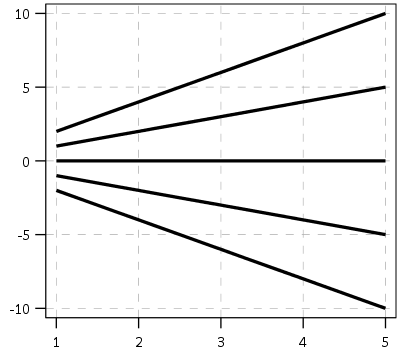This points to an important assumption about those lines not crossing though that was never mentioned in the Barnes paper — the domain matters. For instance, if we draw those rays further back in time what happens?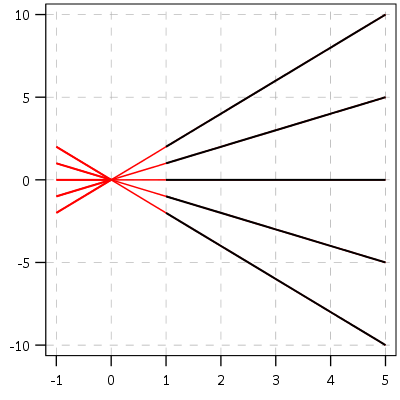They cross! This points to an important piece of information when evaluating PChange — the temporal domain in which you examine the data matters. So if you have a sample of juvenile delinquency measures from 14-18 you would find less change than a similar sample from 12-20.

This isn’t really a critique of PChange — it is totally reasonable to only want to examine changes within a specific domain. Who cares if delinquency trajectories cross when people are babies! But it should be an important piece of information researchers use in the future if they use PChange — longer samples will show more change. It also won’t be fair to compare PChange for samples of different lengths.

# A Functional Approach to PChange

For above you may ask — how would you tell if a trajectory crosses outside of the domain of the data? The answer to that question is you estimate an underlying function of the trajectory — some type of model where the outcome is a function of age (or time). With that function you can estimate the trajectory going back in time or forward in time (or in between sampled measurements). You may not want to rely on data outside of the domain (its standard error will be much higher than data within the time domain, forecasting is always fraught with peril!), but the domain of your sample is ultimately arbitrary. So what about the question will the trajectories ever cross? Or would the trajectories have crossed if I had data for ages 12-20 instead of just 16-18? Or would they have crossed if I checked the juveniles at age 16 1/2 instead of only at 16?

So actually instead of the original way the Barnes paper formulated PChange, here is how I thought about calculating PChange. First you estimate the underlying trajectory for each individual in your sample, then you take the difference of those trajectories.

``````y_i = f(t)
y_j = g(t)
y_delta = f(t) - g(t) = d(t)``````

Where `y_i` is the outcome `y` for observation `i`, and `y_j` is the outcome `y` for observation `j`. `t` is a measure of time, and thus the anonymous functions `f` and `g` represent growth models for observations i and j over time. `y_delta` is then the difference between these two functions, which I represent as the new function `d(t)`. So for example the functions for each individual might be quadratic in time:

``````y_i = b0i + b1i(t) + b2i(t^2)
y_j = b0j + b1j(t) + b2j(t^2)``````

Subsequently the difference function will also be quadratic, and can be simply represented as:

``y_delta = (b0i - b0j) + (b1i - b1j)*t + (b2i - b2j)*t^2``

Then for the trajectories to cross (or at least touch), `y_delta` just then has to equal zero at some point along the function. If this were math, and the trajectories had no errors, you would just set d(t) = 0 and solve for the roots of the equation. (Most people estimating models like these use functions that do have roots, like polynomials or splines). If you cared about setting the domain, you would then just check if the roots are within the domain of interest — if they are, the trajectories cross, if they are not, then they do not cross. For data on humans with age, obviously roots for negative human years will not be of interest. But that is a simple way to solve the domain problem – if you have an underlying estimate of the trajectory, just see how often the trajectories cross within equivalent temporal domains in different samples.

I’d note that the idea of having some estimate of the underlying trajectory is still relevant even within the domain of the data — not just extrapolating to time periods outside. Consider two simple curves below, where the points represent the time points where each individual was measured.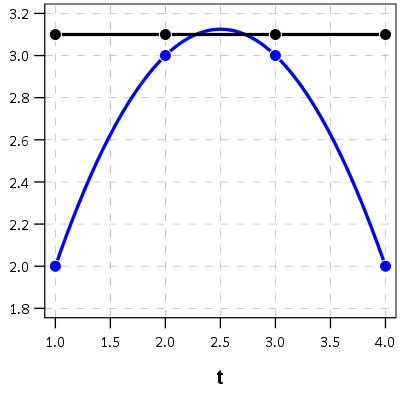So while the two functions cross, when only considering the sampled locations, like is done in Barnes et al.’s PChange, you would say these trajectories do not cross, when in actuality they do. It is just the sampled locations are not at the critical point in the example for these two trajectories.

This points to another piece of contextual information important to interpreting PChange — the number of sample points matter. If you have samples every 6 months, you will likely find more changes than if you just had samples every year.

I don’t mean here to bag on Barnes original metric too much — his PChange metric does not rely on estimating the underlying functional form, and so is a non-parametric approach to identifying change. But estimating the functional form for each individual has some additional niceties — one is that you do not need the measures to be at equivalent sample locations. You can compare someone measured at 11, 13, and 18 to someone who is measured at 12, 16, and 19. For people analyzing stuff for really young kids I bet this is a major point — the underlying function at a specific age is more important then when you conveniently measured the outcome. For older kids though I imagine just comparing the 12 to 11 year old (but in the same class) is probably not a big deal for delinquency. It does make it easier though to compare say different cohorts in which the measures are not at nice regular intervals (e.g. Add Health, NLYS, or anytime you have missing observations in a longitudinal survey).

In the end you would only want to estimate an underlying functional form if you have many measures (more so than 3 in my example), but this typically ties in nicely with what people modeling the behavior over time are already doing — modeling the growth trajectories using some type of functional form, whether it is a random effects model or a group based trajectory etc., they give you an underlying functional form. If you are willing to assume that model is good enough to model the trajectories over time, you should think it is good enough to calculate PChange!

# The Null Matters

So this so far would be fine and dandy if we had perfect estimates of the underlying trajectories. We don’t though, so you may ask, even though `y_delta` does not exactly equal zero anywhere, its error bars might be quite wide. Wouldn’t we then still infer that there is a high probability the two trajectories cross? This points to another hidden assumption of Barnes PChange — the null matters. In the original PChange the null is that the two trajectories do not cross — you need a sufficient change in consecutive time periods relative to the standard error to conclude they cross. If the standard error is high, you won’t consider the lines to cross. Consider the simple table below:

``````Period A_Level A_SE B_Level B_SE
1      4         1    1.5   0.5
2      5         1    3     0.5
3      6         1    4.5   0.5
4      7         1    6     0.5``````

Where A_Level and B_Level refer to the outcome for the four time periods, and A_SE and B_SE refer to the standard errors of those measurements. Here is the graph of those two trajectories, with the standard error drawn as areas for the two functions (only plus minus one standard error for each line).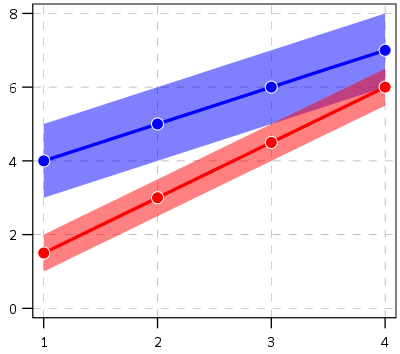And here is the graph of the differences — assuming that the covariance between the two functions is zero (so the standard error of the difference equals `sqrt(A_SE^2 + B_SE^2)`). Again only plus/minus one standard error.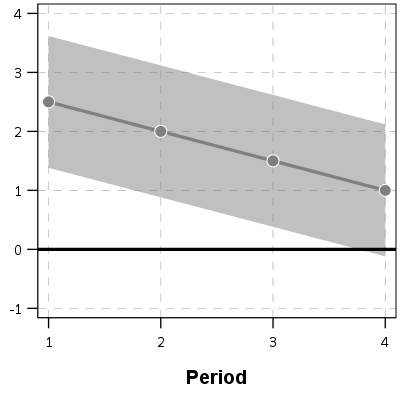You can see that the line never crosses zero, but the standard error area does. If our null is `H0: y_delta = 0 for any t`, then we would fail to reject the null in this example. So in Barnes original PChange these examples lines would not cross, whereas with my functional approach we don’t have enough data to know they don’t cross. This I suspect would make a big difference in many samples, as the standard error is going to be quite large unless you have very many observations and/or very many time points.

If one just wants a measure of crossed or did not cross, with my functional approach you could set how wide you want to draw your error bars, and then estimate whether the high or low parts of that bar cross zero. You may not want a discrete measure though, but a probability. To get that you would integrate the probability over the domain of interest and calculate the chunk of the function that cross zero. (Just assume the temporal domain is uniform across time.)

So in 3d, my difference function would look like this, whereas to the bottom of the wall is the area to calculate the probability of the lines crossing, and the height of the surface plot is the PDF at that point. (Note the area of the density is not normalized to sum to 1 in this plot.)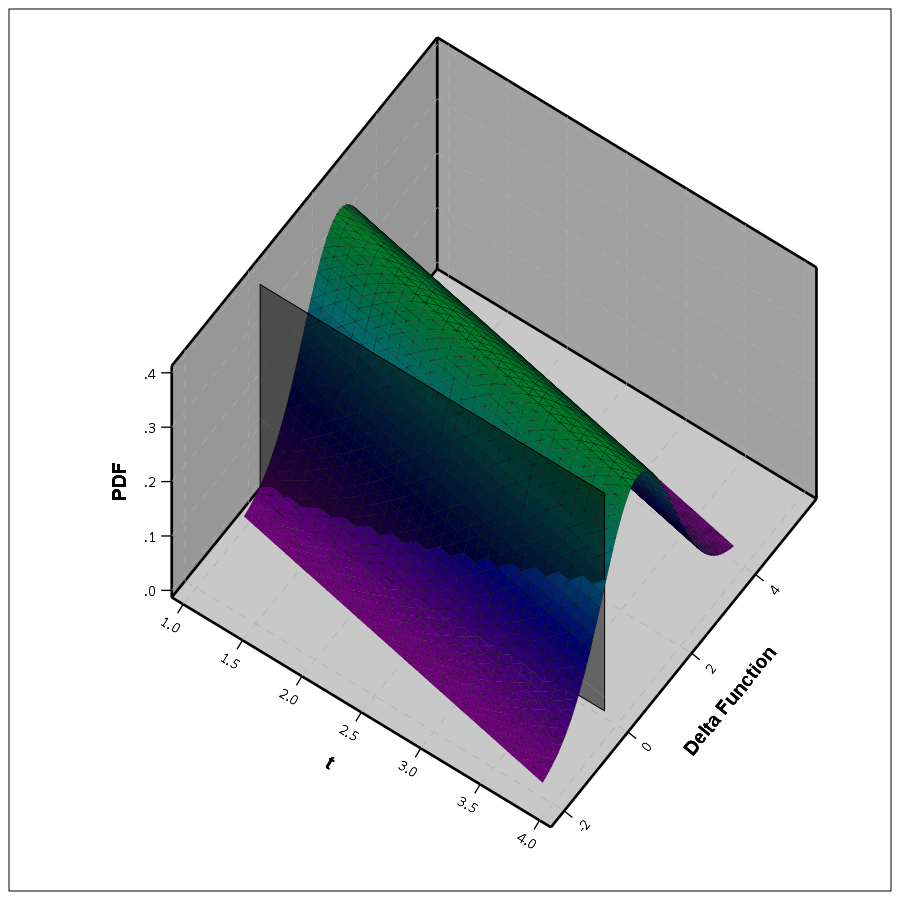This surface graph ends up crossing more than was observed in my prior 2d plots, as I was only plotting 1 standard error. Here imagine that the top green part of the density is the mean function — which does not cross zero — but then you have a non-trivial amount of the predicted density that does cross the zero line.

In the example where it just crosses one time by a little, it seems obvious to consider the small slice as the probability of the two lines crossing. I think to extend this to not knowing to test above or below the line you could calculate the probability on either side of the line, take the minimum, and then double that minimum for your p-value. So if say 5% of the area is below the line in my above example, you would double it and say the two-tailed p-value of the lines crossing is p = 0.10. Imagine the situation in which the line mostly hovers around 0, so the mass is about half on one side and half on the other. In that case the probability the lines cross seems much higher than 50%, so doubling seems intuitively reasonable.

So if you consider this probability to be a p-value, with a very small p-value you would reject the null that the lines cross. Unlike most reference distributions for p-values though, you can get a zero probability estimate of the lines crossing. You can aggregate up those probabilities as weights when calculating the overall PChange for the sample. So you may not know for certain if two trajectories cross, but you may be able to say these two trajectories cross with a 30% probability.

Again this isn’t to say that PChange is bad — it is just different. I can’t give any reasoning whether assuming they do cross (my functional approach) or assuming they don’t cross (Barnes PChange) is better – they are just different, but would likely make a large difference in the estimated number of crossings.

# Population Change vs Individual Level Change

So far I have just talked about trying to determine whether two individual lines cross. For my geographic analysis of trajectories in which I have the whole population (just a sample in time), this may be sufficient. You can calculate all pairwise differences and then calculate PChange (I see no data based reason to use the permutation sample approach Barnes suggested – we don’t have that big of samples, we can just calculate all pairwise combinations.)

But for many of the life course researchers, they are more likely to be interested in estimating the population of changes from the samples. Here I will show how you can do that for either random effects models, or for group based trajectory models based on the summary information. This takes PChange from a sample metric to a population level metric implied via your models you have estimated. This I imagine will be much easier to generalize across samples than the individual change metrics, which will be quite susceptible to outlier trajectories, especially in small samples.

First lets start with the random effects model. Imagine that you fit a linear growth model — say the random intercept has a variance of 2, and the random slope has a variance of 1. So these are population level metrics. The fixed effects and the covariance between the two random effect terms will be immaterial for this part, as I will discuss in a moment.

First, trivially, if you selected two random individuals from the population with this random effects distribution, the probability their underlying trajectories cross at some point is 1. The reason is for linear models, two lines only never cross if the slopes are perfectly parallel. Which sampling from a continuous random distribution has zero probability of them being exactly the same. This does not generalize to more complicated functions (imagine parabolas concave up and concave down that are shifted up and down so they never cross), but should be enough of a motivation to make the question only relevant for a specified domain of time.

So lets say that we are evaluating the trajectories over the range `t = [10,20]`. What is the probability two individuals randomly sampled from the population will cross? So again with my functional difference approach, we have

``````y_i = b0i + b1i*t
y_j = b0j + b1j*t
y_delta = (b0i - b0j) + (b1i - b1j)*t``````

Where in this case the `b0` and `b1` have prespecified distributions, so we know the distribution of the difference. Note that in the case with no covariates, the fixed effects will cancel out when taking the differences. (Generalizing to covariates is not as straightforward, you could either assume they are equal so they cancel out, or you could have them vary according to additional distributions, e.g. males have an 90% chance of being drawn versus females have a 10% chance, in that case the fixed effects would not cancel out.) Here I am just assuming they cancel out. Additionally, taking the difference in the trajectories also cancels out the covariance term, so you can assume the covariance between `(b0i - b0j)` and `(b1i - b1j)` is zero even if `b0` and `b1` have a non-zero covariance for the overall model. (Post is long enough — I leave that as an exercise for the reader.)

For each of the differences the means will be zero, and the variance will be the two variances added together, e.g. `b0i - b0j` will have a mean of zero and a variance of `2 + 2 = 4`. The variance of the difference in slopes will then be 2. Now to figure out when the two lines will cross.

If you make a graph where the X axis is the difference in the intercepts, and the Y axis is the difference in the slopes, you can then mark off areas that indicate the two lines will cross given the domain. Here for example is a sampling of where the lines cross – red is crossing, grey is not crossing.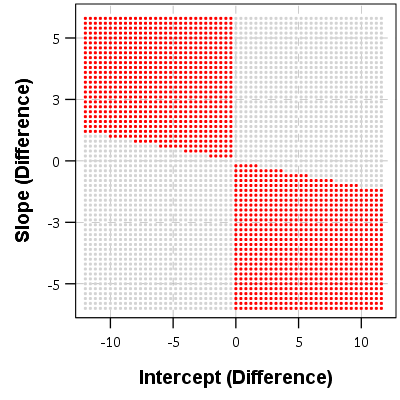So for example, say we had two random draws:

``````y_i = 1   + 0.5*t
y_j = 0.5 + 0.3*t
y_delta = 0.5 + 0.2*t``````

This then shows that the two lines do not cross when only evaluating `t` between 10 and 20. They have already diverged that far out (you would need negative `t` to have the lines cross). Imagine if `y_delta = -6 + 0.2*t` though, this line does cross zero though, at `t = 10` this function equals -1, whereas at `t = 20` the function equals 4.

If you do another 3d plot you can plot the bivariate PDF. Again integrate the chunks of areas in which the function crosses zero, and voila, you get your population estimate.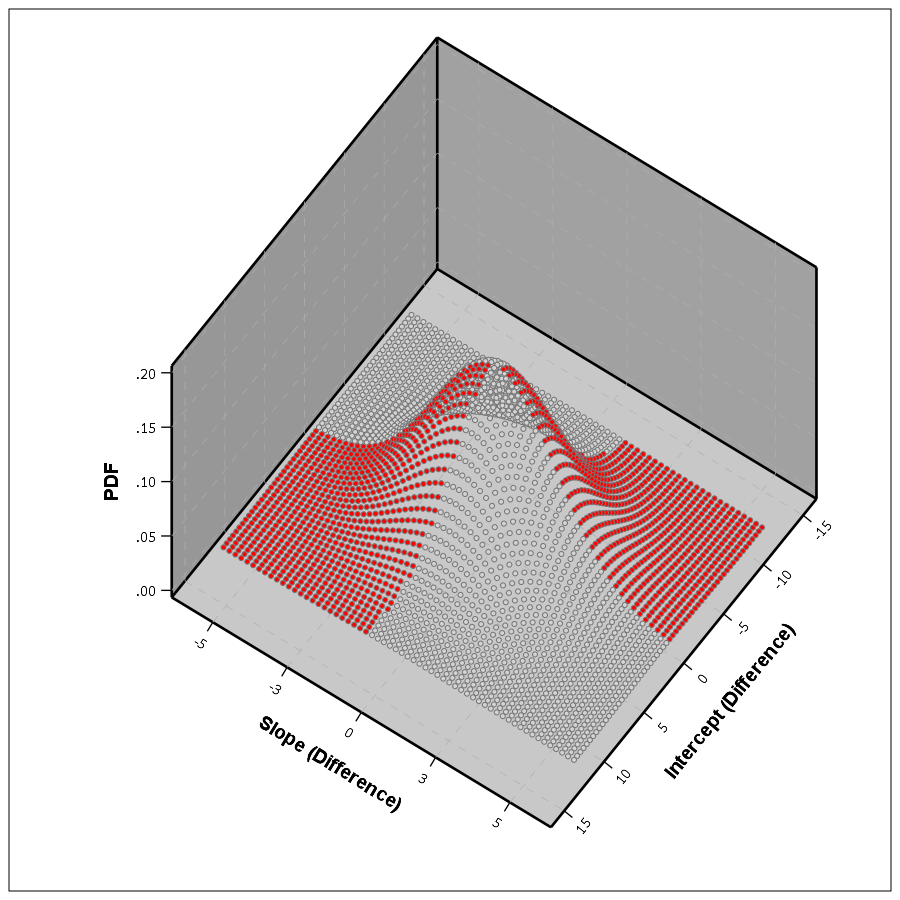This works in a similar manner to higher order polynomials, but you can’t draw it in a nice graph though. I’m blanking at the moment of a way to find these areas offhand in a nice way — suggestions welcome!

This gets a bit tricky thinking about in relation to individual level change. This approach does not assume any error in the random draws of the line, but assumes the draws will have a particular distribution. So the PChange does not come from adding up whether individual lines in your sample cross, it comes from the estimated distribution of what the difference in two randomly drawn lines would look like that is implied by your random effects model. Think if based on your random effect distribution you randomly drew two lines, calculated if they crossed, and then did this simulation a very large number of times. The integrations I’m suggesting are just an exact way to calculate PChange instead of the simulation approach.

If you were to do individual change from your random effects model you would incorporate the standard error of the estimated slope and intercept for the individual observation. This is for your hypothetical population though, so I see no need to incorporate any error.

Estimating population level change from group based trajectory models via my functional approach is more straightforward. First, with my functional approach you would assume individuals who share the same latent trajectory will cross with a high probability, no need to test that. Second, for testing whether two individual trajectories cross you would use the approach I’ve already discussed around individual lines and gain the p-value I mentioned.

So for example, say you had a probability of 25% that a randomly drawn person from group A would cross a randomly drawn person from Group B. Say also that Group A has 40/100 of the sample, and Group B is 60/100. So then we have three different groups: A to A, B to B, and A to B. You can then break down the pairwise number in each group, as well as the number of crosses below.

``````Compare   N    %Cross Cross
A-A      780    100    780
B-B     1770    100   1770
A-B     2400     25    600
Total   4950     64   3150``````

So then we have a population level p-change estimate of 64% from our GBTM. All of these calculations can be extended to non-integers, I just made them integers here to simplify the presentation.

Now, that overall PChange estimate may not be real meaningful for GBTM, as the denominator includes pairwise combinations of folks in the same trajectory group, which is not going to be of much interest. But just looking at the individual group solutions and seeing what is the probability they cross could be more informative. For example, although Barnes shows the GBTM models in the paper as not crossing, depending on how wide the standard errors of the functions are (that aren’t reported), this functional approach would probably assign non-zero probability of them crossing (think low standard error for the higher group crossing a high standard error for the low group).

Phew — that was a long post! Let me know in the comments if you have any thoughts/concerns on what I wrote. Simple question — whether two lines cross — not a real simple solution when considering the statistical nature of the question though. I can’t be the only person to think about this though — if you know of similar or different approaches to testing whether two lines cross please let me know in the comments.

# Transforming KDE estimates from Logistic to Probability Scale in R

The other day I had estimates from several logistic regression models, and I wanted to superimpose the univariate KDE’s of the predictions. The outcome was fairly rare, so the predictions were bunched up at the lower end of the probability scale, and the default kernel density estimates on the probability scale smeared too much of the probability outside of the range.

It is a general problem with KDE estimates, and there are two general ways to solve it:

• truncate the KDE and then reweight the points near the edge (example)
• estimate the KDE on some other scale that does not have a restricted domain, and then transform the density back to the domain of interest (example)

The first is basically the same as edge correction in spatial statistics, just in one dimension instead of the two. Here I will show how to do the second in R, mapping items on the logistic scale to the probability scale. The second linked CV post shows how to do this when using the log transformation, and here I will show the same with mapping logistic estimates (e.g. from the output of a logistic regression model). This requires the data to not have any values at 0 or 1 on the probability scale, because these will map to negative and positive infinity on the logistic scale.

In R, first define the logit function as `log(p/(1-p)` and the logistic function as `1/(1+exp(-x))` for use later:

``````logistic <- function(x){1/(1+exp(-x))}
logit <- function(x){log(x/(1-x))}``````

We can generate some fake data that might look like output from a logistic regression model and calculate the density object.

``````set.seed(10)
x <- rnorm(100,0,0.5)
l <- density(x)  #calculate density on logit scale``````

This blog post goes through the necessary math, but in a nut shell you can’t simply just transform the density estimate using the same function, you need to apply an additional transformation (referred to as the Jacobian). So here is an example transforming the density estimate from the logistic scale, `l` above, to the probability scale.

``````px <- logistic(l\$x)  #transform density to probability scale
py <- l\$y/(px*(1-px))
plot(px,py,type='l')``````

To make sure that the area does sum to one, we can superimpose the density calculated on the data transformed to the probability scale. In this example of fake data the two are pretty much identical. (Black line is my transformed density, and the red is the density estimate based on the probability data.)

``````dp <- density(logistic(x)) #density on the probability values to begin with
lines(dp\$x,dp\$y,col='red')``````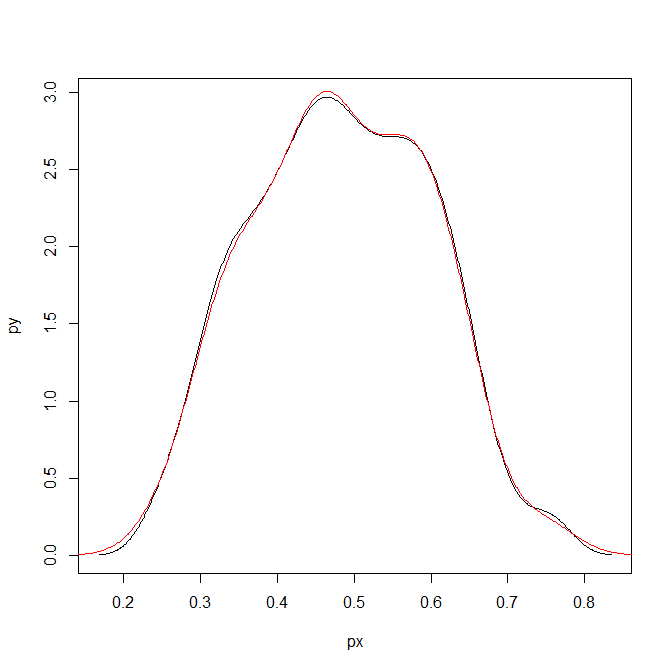Here is a helper function, `denLogistic`, to do this in the future, which simply takes the data (on the logistic scale) and returns a density object modified to the probability scale.

``````logistic <- function(x){1/(1+exp(-x))}
logit <- function(x){log(x/(1-x))}
denLogistic <- function(x){
d <- density(x)
d\$x <- logistic(d\$x)
d\$y <- d\$y/(d\$x*(1-d\$x))
d\$call <- 'Logistic Density Transformed to Probability Scale'
d\$bw <- paste0(signif(d\$bw,4)," (on Logistic scale)")
return(d)
}``````

In cases where more of the probability density is smeared beyond 0-1 on the probability scale, the logistic density estimate will look different. Here is an example with a wider variance and more predictions near zero, so the two estimates differ by a larger amount.

``````lP <- rnorm(100,-0.9,1)
test <- denLogistic(lP)
plot(test)
lines(density(logistic(lP)),col='red')``````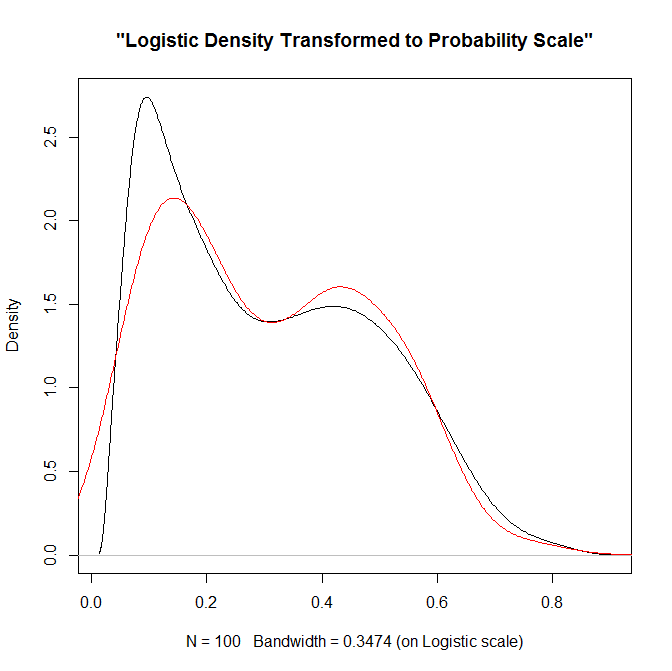Again, this works well for data on the probability scale that can not be exactly zero or one. If you have data like that, the edge correction type KDE estimators are better suited.